# Compare

Compare with characters >, <, =:

85.5780.83

#### Solution:

$85.57>80.83$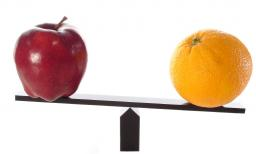We would be pleased if you find an error in the word problem, spelling mistakes, or inaccuracies and send it to us. Thank you!## Next similar math problems:

• Compare IIWhich of the numbers 710, 107 is greater?
• Compare: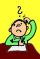What is bigger? ?
• Circumscribed circle to squareFind the length of a circle circumscribing a square of side 10 cm. Compare it to the perimeter of this square.
• Variability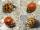In the table bellow the number of wrong produced goods in two shifts: morning shift: 2; 0; 6; 10; 2; 2; 4; 2; 5; 2; afternoon shift: 4; 4; 0; 2; 10; 2; 6; 2; 3; 10; Compare the variability in both shifts, compare the average number of wrong goods on both
• CompareCompare fractions ?. Which fraction of the lower?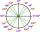What is greater angle? -0.7 radians or -0.7π radians?
• Identity123456789 = 100 Use only three plus or minus characters to correct previous identity/equation.
• Computer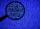A line of print on a computer contains 64 characters (letters, spacers or other chars). Find how many characters there are in 7 lines.
• Cheaper cars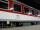State-owned railway company buys 10 air-conditioned coaches for nearly 18 million euros. Calculate how many euros is the equivalent of one seat in the wagon with a capacity of 83 people. How many of used cars at the price 2700 Euros can be buy instead of
• Table 3x3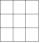Table with numbers 3x3 in the first row 66,24,33 in the second row 57,? 19 in the third row 18,45,60 What number comes ar mark ?. Maybe the numbers: 22,46,45,47
• The door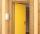The door to the apartment has a height 2 m and a width 80 cm. Calculate how many cm of sealing tape is needed to seal them. Will there be enough packaging in which there are 5 m tape (write 0 = no, 1 = yes)?
• Inclined plane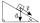1. How much work W we have to do to pull a body weighing 200 kg along an inclined plane with a length of 4 m to a total height of 1.5 m. 2. Find the force we need to exert to do this if we neglect frictional resistance. 3. Find the force we would need if
• Sphere floatingWill float a hollow iron ball with an outer diameter d1 = 20cm and an inside diameter d2 = 19cm in the water? The iron density is 7.8 g/cm 3. (Instructions: Calculate the average sphere density and compare with the density of the water. )
• Triangle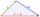Determine if it is possible to construct a triangle with sides 28 31 34 by calculation.
• Compare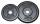What's more? Fill in the signs of equality or inequality: 12 000mg ? 0,12kg 12t ? 0,12kg 14kg ? 0,014t 423mg ? 0,423kg 12,3g ? 0,001 23kg 89g ? 89 000kg
• Foot area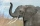Which animal will leave deeper footstep: an elephant weighing 5 tons with a total foot area of 0.5 square meters, or a gazelle weighing 10 kg and a foot area of 50 cm square?
• Angles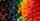Which of those angles are obtuse?